Algebra 2 Worksheets

Dynamically Created Algebra 2 Worksheets

Here is a graphic preview for all of the Algebra 2 Worksheet Sections. You can select different variables to customize these Algebra 2 Worksheets for your needs. The Algebra 2 Worksheets are randomly created and will never repeat so you have an endless supply of quality Algebra 2 Worksheets to use in the classroom or at home. We have fifteen different topics covering Basic Skills, Complex Numbers, Conic Sections, Equations and Inequalities, Exponential and Logarithmic Functions, General Functions, Linear Functions, Matrices, Polynomial Functions, Quadratic Functions and Inequalities, Radical Functions and Rational Exponents, Rational Expressions, Sequences and Series, and Systems of Equations and Inequalities for your use.

Our Algebra 2 Worksheets are free to download, easy to use, and very flexible.

These Algebra 2 Worksheets are a good resource for students in the 9th Grade through the 12th Grade.

Click here for a Detailed Description of all the Algebra 2 Worksheet Sections.

Quick Link for All Algebra 2 Worksheet Sections

Click the image to be taken to that Algebra 2 Worksheet Section.

Basic SkillsAlgebra 2 Worksheets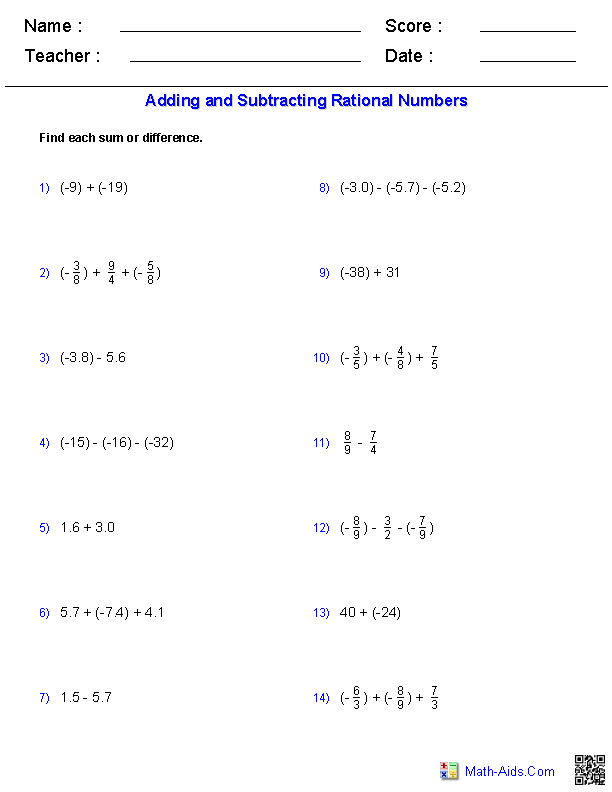Complex Numbers WorksheetsAlgebra 2 Worksheets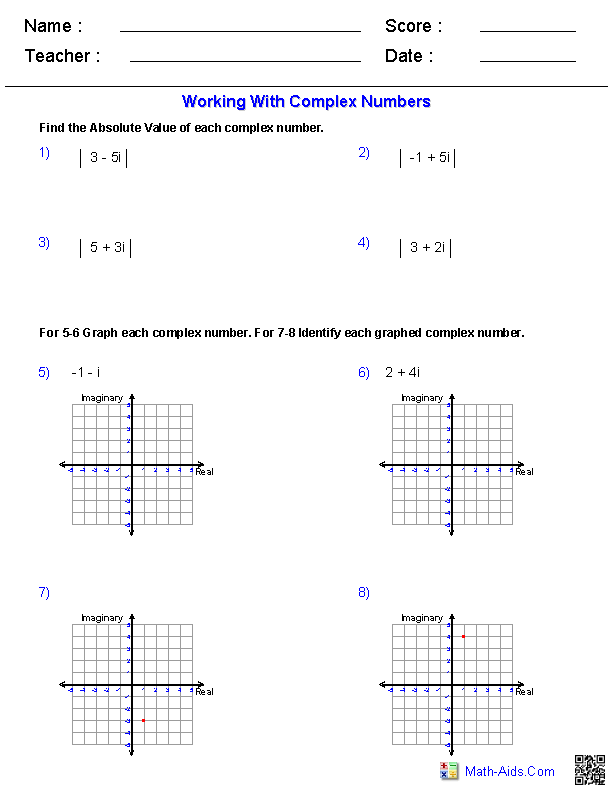Conic Sections WorksheetsAlgebra 2 WorksheetsEquations and InequalitiesAlgebra 2 Worksheets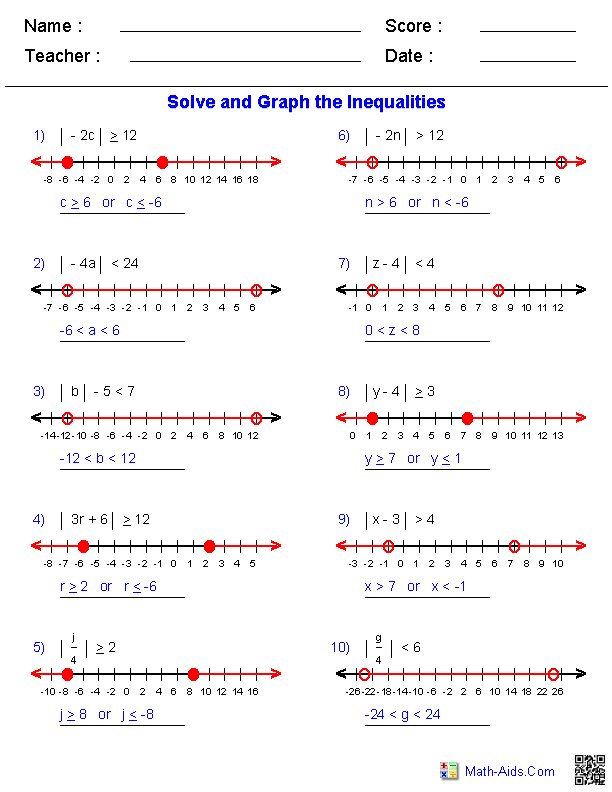Exponential and LogarithmicFunctionsAlgebra 2 Worksheets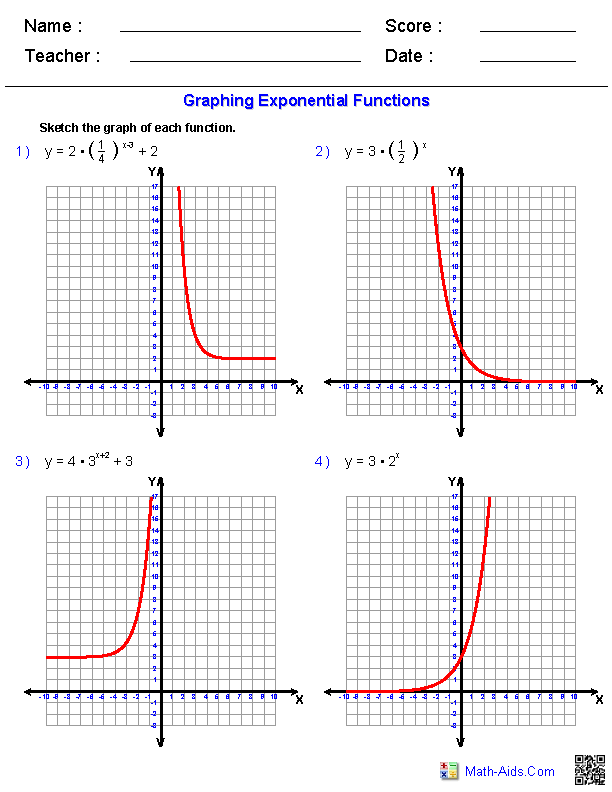General FunctionsAlgebra 2 Worksheets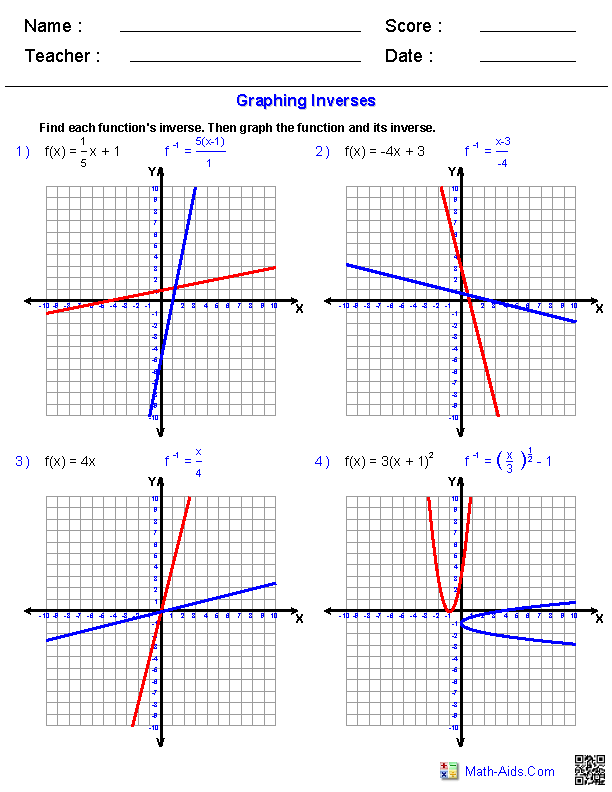Linear FunctionsAlgebra 2 Worksheets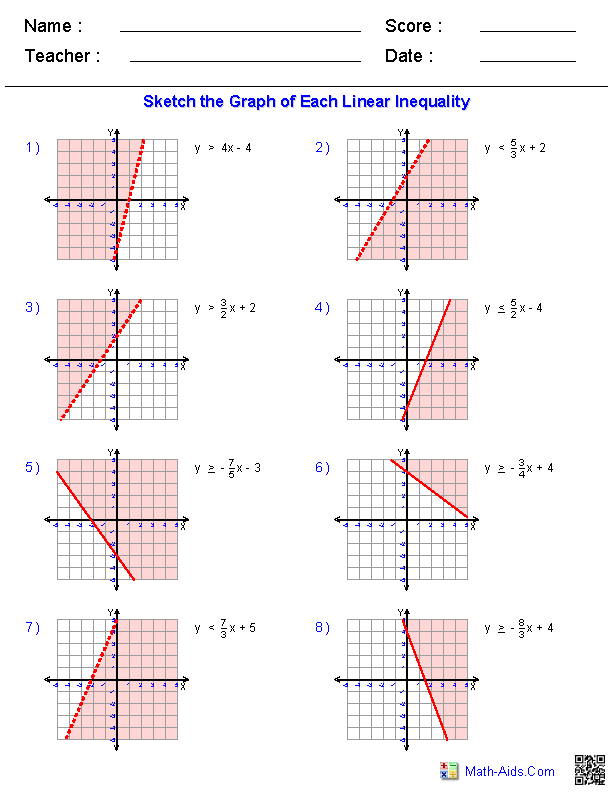Matrices WorksheetsAlgebra 2 WorksheetsPolynomial FunctionsAlgebra 2 WorksheetsQuadratic Functionsand InequalitiesAlgebra 2 Worksheets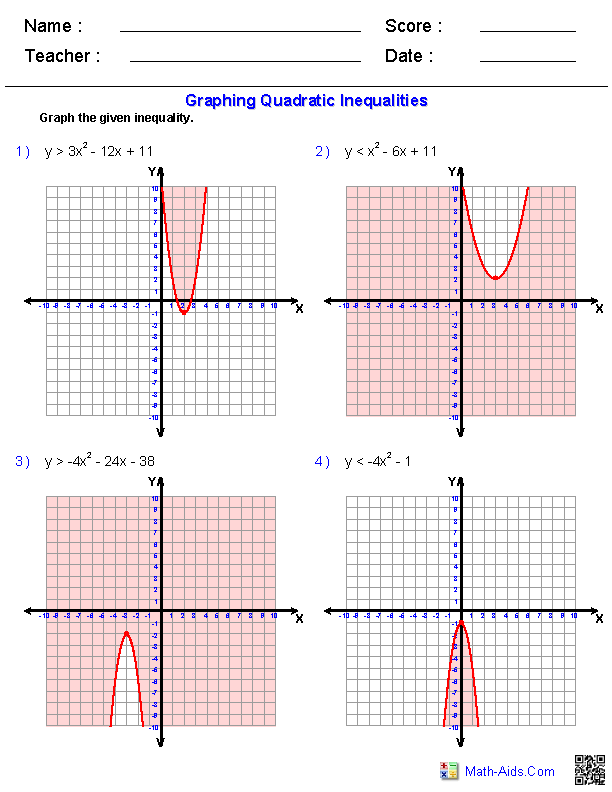Radical FunctionsAlgebra 2 WorksheetsRational ExpressionsAlgebra 2 WorksheetsSequences and SeriesAlgebra 2 Worksheets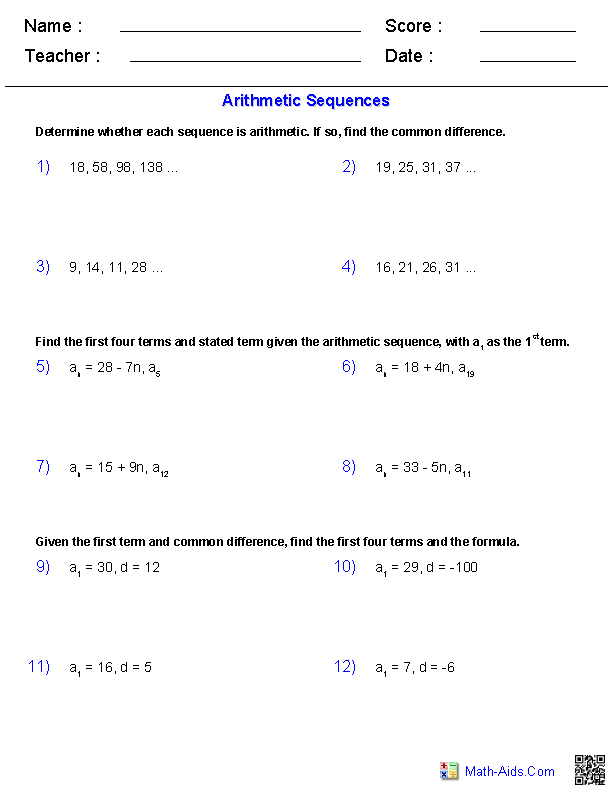Systems of Equationsand InequalitiesAlgebra 2 WorksheetsRecommended Videos

Detailed Description for All Algebra 2 Worksheet Sections

Basic Skills Worksheets
This section contains all of the graphic previews for the Basics for Algebra 2 Worksheets. We currently have worksheets covering order of operations, evaluating expressions, adding and subtracting rational numbers, and multiplying and dividing rational numbers. These Basics for Algebra 2 Worksheets are a good resource for students in the 9th Grade through the 12th Grade.

Complex Numbers Worksheets
This section contains all of the graphic previews for the Complex Numbers Worksheets. We currently have worksheets covering handout for complex numbers, finding the absolute value of complex numbers, graphing and writing equations for complex numbers, working with complex numbers, operations with complex numbers, and rationalizing imaginary denominators. These Complex Numbers for Algebra 2 Worksheets are a good resource for students in the 9th Grade through the 12th Grade.

Conic Sections Worksheets
This section contains all of the graphic previews for the Conic Sections Worksheets. We currently have worksheets covering graphing and properties of parabolas, equations of parabolas, graphing and properties of circles, equations of circles, graphing and properties of ellipses, equations of ellipses, graphing and properties of hyperbolas, equations of hyperbolas, classifying conic sections, eccentricity, and systems of quadratic equations. These Conic Sections for Algebra 2 Worksheets are a good resource for students in the 9th Grade through the 12th Grade.

Equations and Inequalities Worksheets
This section contains all of the graphic previews for the Equations and Inequalities Worksheets. We currently have worksheets covering multi-step equations with integers, decimals, and fractions. We have absolute value equations, multi-step inequalities, compound inequalities, and absolute value inequalities. We have several word problem in this section such as two step equations, "distance, rate, and time" problems, mixture problems, and work word problems. These Equations and Inequalities Worksheets are a good resource for students in the 9th Grade through the 12th Grade.

Exponential and Logarithmic Functions Worksheets
This section contains all of the graphic previews for the Exponential and Logarithmic Functions Worksheets. We currently have worksheets covering the meaning of logarithms, properties of logarithms, the change of base formula, writing logs in terms of others, logarithmic equations, inverse functions and logarithms, exponential equations with and without logarithms, graphing logarithms, and graphing exponential functions. These Exponential Functions Worksheets are a good resource for students in the 9th Grade through the 12th Grade.

General Functions Worksheets
This section contains all of the graphic previews for the General Functions Worksheets. We currently have worksheets covering evaluating functions, function operators, and inverse functions. These General Functions Worksheets are a good resource for students in the 9th Grade through the 12th Grade.

Linear Functions Worksheets
This section contains all of the graphic previews for the Linear Functions Worksheets. We currently have worksheets covering review of linear equations, graphing absolute value functions, graphing linear inequalities. These Linear Functions Worksheets are a good resource for students in the 9th Grade through the 12th Grade.

Matrices Worksheets
This section contains all of the graphic previews for the Matrices Worksheets. We currently have worksheets covering basic matrix operations, matrix multiplication, all matrix operations combined, determinants, matrix inverses, Cramer's rule, matrix equations, geometric transformations with matrices. These Matrices Worksheets are a good resource for students in the 9th Grade through the 12th Grade.

Polynomial Functions Worksheets
This section contains all of the graphic previews for the Polynomial Functions Worksheets. We currently have worksheets covering naming polynomials, factoring, The Remainder Theorem, Irrational and Imaginary Root Theorems, Descartes' Rule of Signs, The Rational Root Theorem, polynomial equations, basic shapes and graphs of polynomials, graphing polynomial functions, and The Binomial Theorem. These Polynomial Functions Worksheets are a good resource for students in the 9th Grade through the 12th Grade.

This section contains all of the graphic previews for the Quadratic Functions Worksheets. We currently have worksheets covering properties of parabolas, vertex form, graphing quadratic inequalities, factoring quadratic expressions, solving quadratic equations, completing the square, solving equations by completing the square, solving equations with the quadratic equation, and the discriminant. These Quadratic Functions Worksheets are a good resource for students in the 9th Grade through the 12th Grade.

This section contains all of the graphic previews for the Radical Functions Worksheets. We currently have worksheets covering simplifying radicals, operations with radical expressions, dividing radical expressions, radical and rational exponents, simplifying rational exponents, square root equations, rational exponent equations, and graphing radicals. These Radical Functions Worksheets are a good resource for students in the 9th Grade through the 12th Grade.

Rational Expressions Worksheets
This section contains all of the graphic previews for the Rational Expressions Worksheets. We currently have worksheets covering graphing simple rational functions, graphing general rational functions, simplifying rational expressions, adding and subtracting rational expressions, multiplying rational expressions, dividing rational expressions, complex fractions, and solving rational equations. These Rational Expressions Worksheets are a good resource for students in the 9th Grade through the 12th Grade.

Sequences and Series Worksheets
This section contains all of the graphic previews for the Sequences and Series Worksheets. We currently have worksheets covering general sequences, arithmetic sequences, geometric sequences, comparing arithmetic/geometric sequences, general series, arithmetic series, arithmetic/geometric means with sequences, finite geometric series, and infinite geometric series. These Sequences and Series Worksheets are a good resource for students in the 9th Grade through the 12th Grade.

System of Equations and Inequalities Worksheets
This section contains all of the graphic previews for the System of Equations and Inequalities Worksheets. We currently have worksheets covering systems of two linear inequalities, systems of two equations, systems of two equations word problems, points in three dimensions, planes, systems of three equations by elimination, systems of three equations by substitution, and Cramer's Rule. These System of Equations and Inequalities Worksheets are a good resource for students in the 9th Grade through the 12th Grade.